Next: SLA_PRECES - Precession
Up: SUBPROGRAM SPECIFICATIONS
Previous: SLA_PREBN - Precession Matrix (FK4)

## SLA_PREC - Precession Matrix (FK5)

ACTION:
Form the matrix of precession between two epochs (IAU 1976, FK5).
CALL:
CALL sla_PREC (EP0, EP1, RMATP)

GIVEN:

 D EP0 beginning epoch EP1 D ending epoch

RETURNED:

 D(3,3) RMATP precession matrix

NOTES:
1.
The epochs are TDB Julian epochs.
2.
The matrix is in the sense:
v1 = Mv0
where v1 is the star vector relative to the mean equator and equinox of epoch EP1, M is the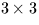matrix RMATP and v0 is the star vector relative to the mean equator and equinox of epoch EP0.
3.
Though the matrix method itself is rigorous, the precession angles are expressed through canonical polynomials which are valid only for a limited time span. There are also known errors in the IAU precession rate. The absolute accuracy of the present formulation is better than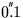from 1960AD to 2040AD, better than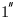from 1640AD to 2360AD, and remains below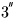for the whole of the period 500BC to 3000AD. The errors exceed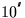outside the range 1200BC to 3900AD, exceed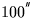outside 4200BC to 5600AD and exceed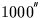outside 6800BC to 8200AD. The SLALIB routine sla_PRECL implements a more elaborate model which is suitable for problems spanning several thousand years.

REFERENCES:
1.
Lieske, J.H., 1979. Astr.Astrophys. 73, 282; equations 6 & 7, p283.
2.
Kaplan, G.H., 1981. USNO circular no. 163, pA2.Next: SLA_PRECES - Precession
Up: SUBPROGRAM SPECIFICATIONS
Previous: SLA_PREBN - Precession Matrix (FK4)

SLALIB --- Positional Astronomy Library